﻿ Calculation method > Indoor method

# INDOOR METHOD

Navegación:  Calculation method > Indoor method

# INDOOR METHOD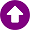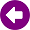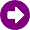DESCRIPTION

It is a semi-empirical model of propagation in indoor environments. The basis for this calculation method is a classic indoor propagation model, which includes losses when passing through walls and floors. Additionally, other additional effects present in these scenarios are considered, such as reflections and diffractions.

The complete model expression for distances less than the break-up distance is: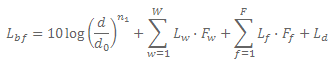(1)

For distances greater than the break-up distance: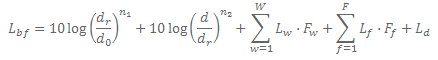(2)

where:

d: Distance in a straight line to the position of the receiver in meters.
d0: Reference distance in meters.
dr: Break-up distance in meters.
n1: Exponent of variation for distances smaller than the break-up distance.
n2: Exponent of variation for distances greater than the break-up distance.
Lw: Losses associated with wall w.
Fw: Factor for the angle of incidence of the direct ray on the wall w.
Lf: Losses associated with the floor f.
Ff: Factor for the angle of incidence of the direct ray on the floor f.
Ld: diffraction losses in walls and corners

DEVELOPMENT

Break-up distance

It is considered that at very close distances to the transmitter, nearby walls and floors do not significantly interact on propagation losses. For this reason, two propagation zones are defined. For distances smaller than the break distance the propagation exponent by distance n1 is used, and for greater distances the exponent n2.

Angle of incidence

When the incidence of the direct ray on the wall or the floor is not completely perpendicular, it is considered that part of the signal is reflected. This effect is included in the model as a factor on the losses produced in transmission through the material. In this way, as the angle of incidence of the ray on the wall or floor is smaller, the transmitted signal is smaller.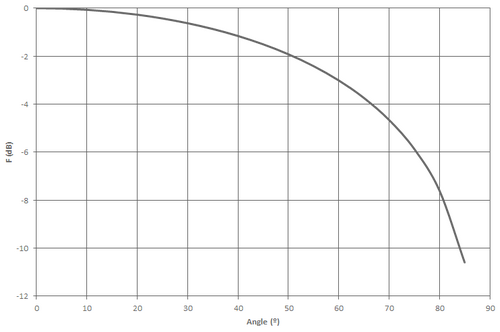Diffraction

The model includes the calculation of diffraction losses. These losses are evaluated taking into account the method proposed for finite conductivity wedges of Rec. ITU-R P.526. These diffraction losses are evaluated both for walls that do not reach the ceiling in the vertical plane, and for corners and walls in the horizontal plane.

The method is based on UTD. It takes into account diffraction in both the shadow and line-of-sight regions and provides a gradual transmission method between these regions.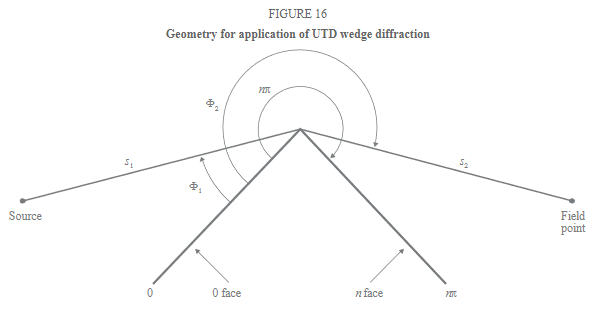REFERENCES

 "A New Empirical Model for Indoor Propagation Prediction". Kwok-Wai Cheung, Jonathan H.-M. Sau, and R. D. Murch, Member, IEEE.

 Recommendation ITU-R P.526-13, "Propagation by diffraction".